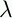September 22, 1999 Perl and the Lambda Calculus Slide #37

# Applicative Order Solution

```        Y =f.(x.(f (x x))x.(f (x x)))
```

Use

```        Y =f.((x.(q.f (x x))q.f (x x))
```

Then when we want to make a recursive call, apply to a dummy argument Q

For example, R becomes:

```g.(mn.(IF (IS_ZERO m) (q.n) (q.(g Q) (PRED m) (SUCC n))) Q)
```

The first Q in there is to force the recursive call if necessary

 NextCopyright © 1999 M-J. Dominus